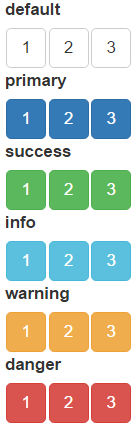# The orderInput

#### 2022-02-03

The orderInput display a list of items and return their order as a vector in shiny input value. You can drag and drop the items to change their display order, and the binding shiny input value will update accordingly. Here is a simple example:

server <- function(input, output) {
output$order <- renderPrint({input$foo})
}

ui <- fluidPage(
orderInput(inputId = 'foo', label = 'A simple example', items = c('A', 'B', 'C')),
verbatimTextOutput('order')
)

shinyApp(ui, server)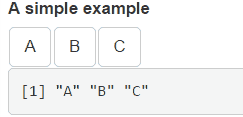The shiny input value input$inputId records the current item order, where the “inputId” is the inputId parameter passed to the function. The items parameter can either be a list, an atomic vector or a factor. • For fully named list and vector (e.g. c(A = "a", B = "b")), orderInput displays their names (A and B) in items and returns their values (a and b) as order. • For unnamed or partial named list and vector (e.g. c("a", B = "b")), the values (a and b) are used for both display and order. • For factor (e.g. factor("a", "b")), the values (a and b) are displayed while their levels (1 and 2) are used as itme order. Multiple orderInputs can be connected to each other by passing their inputIds to the connect parameter. When connected, items from one orderInput can be dragged to another. See the following example: # items in A can be dragged to B orderInput('A', 'A', items = 1:3, connect = 'B') # items in B can be dragged to A orderInput('B', 'B', items = 4:6, connect = 'A')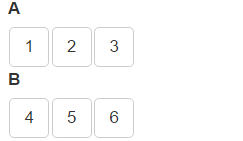A connected orderInput can work in the source mode by setting as_source = TRUE. If an item is dragged from a source-mode-orderInput to other orderInputs, the item will not be removed from the original orderInput, that is, the operation become “copy” instead of “cut”. See the following example: # In source mode, items dragged to B are copied orderInput('A', 'A', items = 1:3, connect = 'B', as_source = TRUE) orderInput('B', 'B', items = 4:6)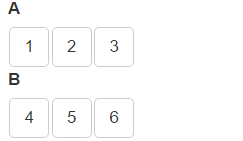Items from non-source orderInput can be deleted by dragging them to a source orderInput: # Anything dropped into a "source" orderInput will be deleted orderInput('A', 'A', items = 1:3, as_source = TRUE), orderInput('B', 'B', items = 4:6)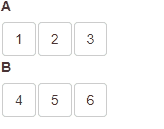orderInput supports the save and load operations and shiny bookmarking. See Vignette Save and restore for more information. Here is an example: ui <- fluidPage( orderInput('A', 'A', items = 1:3, as_source = TRUE, connect = c("B", "C")), orderInput('B', 'B', items = 4:6, connect = "C"), orderInput('C', 'C', items = 7:9, connect = "B"), hr(), actionButton("save", "Save"), actionButton("load", "Load") ) server <- function(input, output, session) { observeEvent(input$save, jqui_sortable("#B,#C", "save"))
}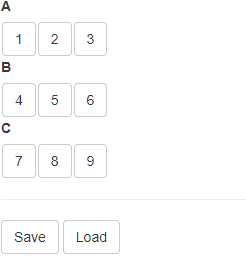placeholder shows when there is no item in an orderInput:

orderInput('A', 'A', items = 1:3, connect = 'B')
orderInput('B', 'B', items = NULL, placeholder = 'Drag item here...')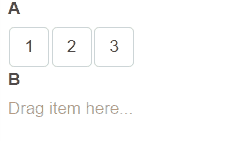orderInput uses the six predefined Bootstrap button classes to style the displayed items. Set it in the item_class parameter:

orderInput('default', 'default', items = 1:3, item_class = 'default')
orderInput('primary', 'primary', items = 1:3, item_class = 'primary')
orderInput('success', 'success', items = 1:3, item_class = 'success')
orderInput('info', 'info', items = 1:3, item_class = 'info')
orderInput('warning', 'warning', items = 1:3, item_class = 'warning')
orderInput('danger', 'danger', items = 1:3, item_class = 'danger')